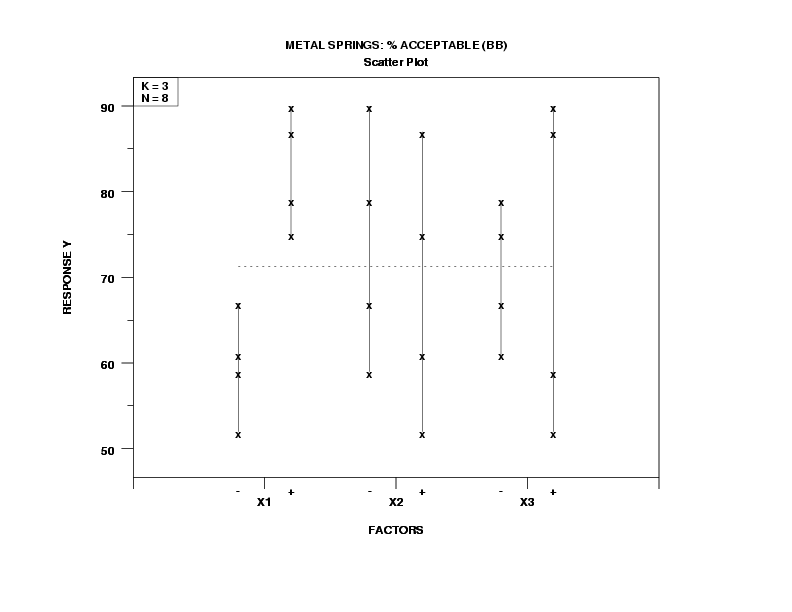# Dex scatter Plots

• Last Updated : 22 Jan, 2021

The scatter plot is used to determine whether two variables have some correlation with each other. This plot uses dots to represent different data points in the dataset. The dex (design and Experiment) scatter plot is a technique used in the analysis of the process.

Dex Scatter plot is used to answer three important questions:

• What data points are outliers? A data point is called outliers if it comes from a different probability distribution or from a different deterministic data point.
• What are the most important factors? Here, the important factors are those which lead to significant variation in the response variable when we change the factor from ‘+’ side to ‘-‘ side or vice-versa.
• What is the best setting for these important factors? The best setting refers to if it results in the response variable achieve the closest to the desired setting or achieve the desired target such as maximum, minimum, etc.

The dex scatters plot consists of the following axes:

• Horizontal axis: The k factors and their positive and negative settings.
• Vertical axis: The output of the response variable for the given set of a factor for each of the k factors.

Below is the graph of the dex scatter plot from this paper.Dex scatter plot of metal rings and percentage of acceptability

Here, we will be answering three questions based on the above :

• Most important factors: Here, we have 3 factors (X1, X2, X3). If we go from ‘-‘ values to ‘+’ setting within the factor, we need to find out which factor is affecting the biggest location shift. That factor is called the ‘most important’ factor, which factor is the second most factor, and so on. Here, the most important factor is X1, then X2 of slightly less important, and X3 of least important.
• The best setting for the most important factors: Now, we have to find which of the settings (‘-‘ or ‘+’) results in a more desirable response. Suppose, we want to maximize the value of the response variable, then we would proceed as follows:
• For factor X1, for what setting (- or +) is the body of the data higher? Similarly, for factor X2 and factor X3. The resulting k-vector of the best settings are:
`(X1_best, X2_best, ..., XK_best)`

The best setting for the above graph is:

`(X1_best, X2_best, X3_best) =  ('+','-','-') = ('+1', '-1', '-1')`
• Outliers: Outliers are the data points which would stand apart from most of the data point. There are no outliers detect in the above graph.

References:

My Personal Notes arrow_drop_up
Recommended Articles
Page :